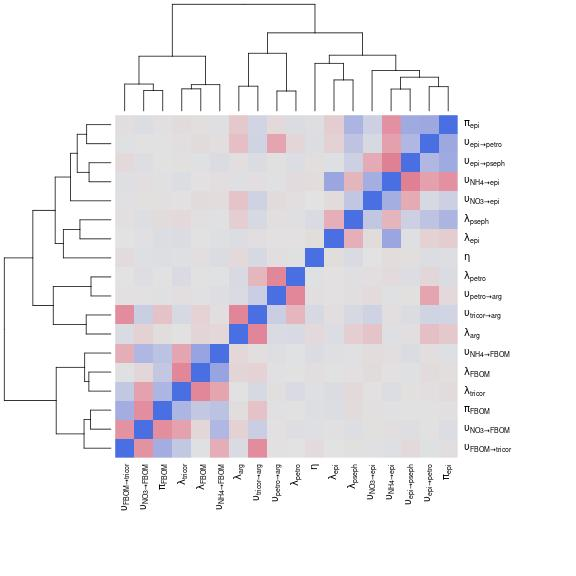# Case study: Trinidadian streams (Collins et al. 2016)

#### 2023-03-20

This case study models nitrogen fluxes at the ecosystem scale from a dataset collected in Trinidadian mountain streams. The data is taken from the following article:

• Collins, S. M., S. A. Thomas, T. Heatherly, K. L. MacNeill, A. O.H.C. Leduc, A. López-Sepulcre, B. A. Lamphere, et al. Fish Introductions and Light Modulate Food Web Fluxes in Tropical Streams: A Whole-Ecosystem Experimental Approach. Ecology (2016) https://doi.org/10.1002/ecy.1530.

The goal is to quantify the nitrogen exchanges in the stream foodweb, from the dissolved nutrients (NH$$_4^+$$ and NO$$_3^-$$) to the top invertebrate predators. Using the isotracer package, we can:

• estimate those fluxes and the associated uncertainties
• test for the existence of suspected trophic links between some species of interest.

## Experiment and data

In brief, $$^{15}N$$-enriched ammonium was dripped in two streams in Trinidad, and samples of the different foodweb compartments were taken during the drip and after the drip in several transects in each stream. The transects were located at different locations downstream of each drip. The drip phase lasted 10 days, and the post-drip phase lasted 30 days. Here is a glimpse of the dataset (we will use only the data from one stream for simplicity, but for a real analysis one could keep both streams and use stream identity as a covariate to estimate the stream effect on flows - see the case study about eelgrasses for an example of treatments comparison):

lalaja
## # A tibble: 247 × 6
## # Groups:   stream, transect 
##    stream transect   compartment mgN.per.m2 prop15N time.days
##    <chr>  <chr>      <chr>            <dbl>   <dbl>     <dbl>
##  1 UL     transect.1 NH4              0.300 0.0240          0
##  2 UL     transect.1 NO3             31.5   0.00397         0
##  3 UL     transect.2 NH4              0.300 0.0140          0
##  4 UL     transect.2 NO3             31.5   0.00407         0
##  5 UL     transect.3 NH4              0.300 0.00694         0
##  6 UL     transect.3 NO3             31.5   0.00413         0
##  7 UL     transect.1 arg              2.31  0.00366         0
##  8 UL     transect.2 arg              2.31  0.00366         0
##  9 UL     transect.3 arg              2.31  0.00366         0
## 10 UL     transect.1 epi            329.    0.00366         0
## # … with 237 more rows
• stream could be one of two locations (LL or UL), but only data from UL is used here.
• transect is nested within stream, and there are three transects per stream.
• compartment is a foodweb compartment.
• time.days is the sampling time.
• mgN.per.m2 is the estimated size of the compartment.
• prop15N is the proportion of $$^{15}$$N in the total nitrogen content of the compartment.

## Building the model

### Network topology

We will only model a simplified version of the foodweb described by Collins et al. to keep the model runtimes short. The foodweb model comprises:

• the two dissolved nutrients (ammonium and nitrate)
• two primary producers (epilithon, the layer of photosynthetic organisms growing on the stream bed, and FBOM, the fine benthic organic matter lying on the stream bed)
• three invertebrate grazers (Petrophila, Psephenus and Tricorythodes).
• one invertebrate predator (Argia), that preys on Petrophila and for which we would like to test another potential trophic link with Psephenus. We will test this extra trophic link later in the vignette.

We initialize the network model with the corresponding topology:

m <- new_networkModel() %>%
set_topo("NH4, NO3 -> epi, FBOM", "epi -> petro, pseph", "FBOM -> tricor",
"petro, tricor -> arg")

Since the inorganic nutrients are being constantly renewed by the stream flow, we will consider them in a steady state. We set both NH4 and NO3 to a steady state in the model:

m <- m %>% set_steady(c("NH4", "NO3"))

This is how our network look like at this stage:

ggtopo(m, "sugiyama")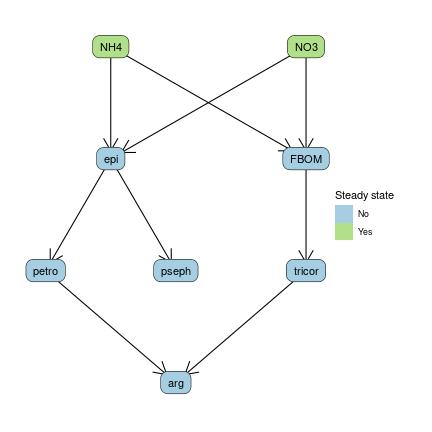### Initial conditions and observations

The drip of $$^{15}N$$-enriched ammonium was started at the very beginning of the experiment, and the initial conditions reflect the enrichment observed for NH4 and NO3 (only ammonium is dripped, but it is converted to nitrate quite rapidly and the experimental setup is thus equivalent to a drip phase for both ions).

The initial conditions are obtained by taking the values when t=0:

library(tidyverse)
inits <- lalaja %>% filter(time.days == 0)

We add them to the model, specifying the grouping variable (transect, each transect being a different location downstream the drip station in the UL stream):

m <- m %>% set_init(inits, comp = "compartment", size = "mgN.per.m2", prop = "prop15N",
group_by = c("transect"))

The replication structure of the model can be examined using the groups() function:

groups(m)
## # A tibble: 3 × 1
##   transect
##   <chr>
## 1 transect.1
## 2 transect.2
## 3 transect.3

The next step is to add the observations made during the experiment:

obs <- lalaja %>% filter(time.days > 0)
m <- m %>% set_obs(obs, time = "time.days")

The enrichment data can be visualized with the plot() method:

# Note the log scale for better visualization
plot(m, facet_row = "group", facet_col = "compartment", type = "prop",
scale = "all", log = TRUE,
comps = c("NH4", "NO3", "epi", "FBOM", "petro", "pseph", "tricor", "arg"))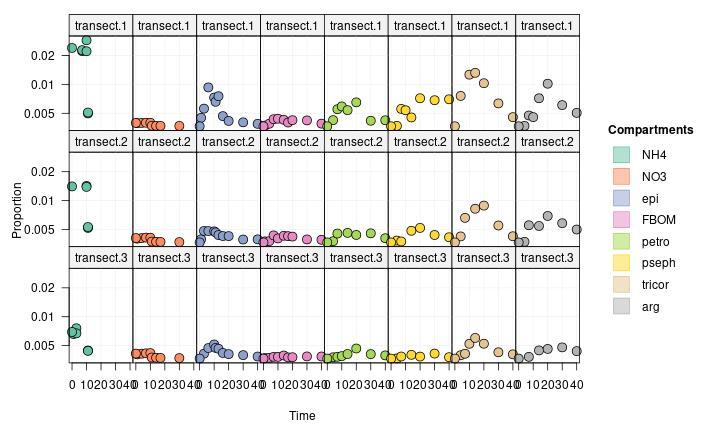Interestingly, we see that one of the consumer, tricor, is over-enriched compared to its resources (epi and FBOM). This is due to the “bulk” nature of the epilithon and FBOM compartments.

### Allowing for inhomogeneous compartments

Some compartment are sampled “in bulk” by the field scientist, but are really comprised of several sub-compartments, which can independently incorporate the marked tracer or be selectively fed upon by a consumer at the time scale of the experiment. For example, “epilithon” is really everything that we can be scratch and collect from the surface of a stream bed rock in the field, but it might actually be composed of an active fraction incorporating the dissolved nutrients (algae growing on the rock) and being selectively grazed by the invertebrate consumers, while the rest is a refractory fraction of sediment and debris which are not involved in the nutrient cycle at the scale of a few days.

To account for that in our model, we can allow for epilithon and FBOM to be split compartments with an active and a refractory portions:

m <- m %>% set_split(c("epi", "FBOM"))

For epi (and similarly for FBOM), this introduces a new parameter, portion.act_epi, which is used to split the initial biomass for the epi compartment into an active portion (involved in the network) and a refractory portion (isolated from the network, but used to predict observed size and proportion marked in the “bulk” epilithon).

### Drip regime

The NH4 and NO3 quantities during the “on” phase of the drip (when the experiment starts) are already defined by (i) the fact that we set ammonium and nitrate to be in a steady state and (ii) the initial conditions. We will specify the quantities for the “off” phase by using the add_pulse_event() function to decrease the amount of $$^{15}N$$ in the ammomiun and nitrate compartments after day 10.

The add_pulse_event() function accepts either a single pulse specification, or bulk pulses defined in a table. We use the table syntax here:

pulses <- tribble(
~ stream,    ~ transect, ~ comp, ~ time, ~ qty_14N, ~ qty_15N,
"UL",  "transect.1",  "NH4",     11,         0,  -0.00569,
"UL",  "transect.2",  "NH4",     11,         0,  -0.00264,
"UL",  "transect.3",  "NH4",     11,         0, -0.000726,
"UL",  "transect.1",  "NO3",     11,         0,  -0.00851,
"UL",  "transect.2",  "NO3",     11,         0,  -0.01118,
"UL",  "transect.3",  "NO3",     11,         0,  -0.01244,
)
m <- m %>% add_pulse_event(pulses = pulses, comp = "comp", time = "time",
unmarked = "qty_14N", marked = "qty_15N")

### Setting the distribution family for observed sizes

Let’s think a moment about the modelling of the observed compartment sizes. By default, the isotracer model assumes that observed compartment pool sizes are distributed following a normal distribution around the values expected from the calculated trajectories, with a coefficient of variation $$\zeta$$ shared by all compartments. This is fine when the compartments exhibit similar variation in their biomasses, but in the case of this ecosystem study they do not.

To illustrate this point, let’s calculate the standard deviations and the corresponding coefficients of variation for the observed compartment sizes:

obs %>% select(compartment, mgN.per.m2) %>%
na.omit() %>%
group_by(compartment) %>%
summarize(mean = mean(mgN.per.m2),
sd = sd(mgN.per.m2),
cv = sd / mean) %>%
arrange(cv)
## # A tibble: 8 × 4
##   compartment     mean      sd    cv
##   <chr>          <dbl>   <dbl> <dbl>
## 1 NH4            0.300    0    0
## 2 NO3           31.5      0    0
## 3 epi          329.     115.   0.350
## 4 FBOM        5267.    2436.   0.463
## 5 pseph         14.4     10.7  0.745
## 6 arg            2.31     2.88 1.25
## 7 tricor         8.38    13.3  1.59
## 8 petro          1.40     2.48 1.77

The coefficients of variation are particularly large for the invertebrates, which is due to the patchiness of their distribution on the stream bed.

One way to handle this in our model is to allow for $$\zeta$$ to be compartment-specific. To make things easier for the model fit here, we will actually provide those compartment-specific $$\zeta$$ values as fixed parameters, but we could also let the model estimate them.

First, we specify that $$\zeta$$ depends on the compartment, and that it represents the standard deviation of the distributions:

m <- m %>% set_size_family("normal_sd", by_compartment = TRUE)

Then, we provide constant priors for those parameters based on the values calculated above:

m <- set_prior(m, constant_p(0.1), "zeta_NH4") %>%  # Dummy values for the steady
set_prior(constant_p(0.1), "zeta_NO3") %>%        # state dissolved nutrients
set_prior(constant_p(115), "zeta_epi") %>%
set_prior(constant_p(2436), "zeta_FBOM") %>%
set_prior(constant_p(10.7), "zeta_pseph") %>%
set_prior(constant_p(2.88), "zeta_arg") %>%
set_prior(constant_p(13.3), "zeta_tricor") %>%
set_prior(constant_p(2.48), "zeta_petro")

## Running the model

### Setting priors

Before we run the model, let’s see which priors we still have to set:

missing_priors(m)
## # A tibble: 20 × 2
##    in_model               prior
##    <chr>                  <list>
##  1 eta                    <NULL>
##  2 lambda_arg             <NULL>
##  3 lambda_epi             <NULL>
##  4 lambda_FBOM            <NULL>
##  5 lambda_NH4             <NULL>
##  6 lambda_NO3             <NULL>
##  7 lambda_petro           <NULL>
##  8 lambda_pseph           <NULL>
##  9 lambda_tricor          <NULL>
## 10 portion.act_epi        <NULL>
## 11 portion.act_FBOM       <NULL>
## 12 upsilon_epi_to_petro   <NULL>
## 13 upsilon_epi_to_pseph   <NULL>
## 14 upsilon_FBOM_to_tricor <NULL>
## 15 upsilon_NH4_to_epi     <NULL>
## 16 upsilon_NH4_to_FBOM    <NULL>
## 17 upsilon_NO3_to_epi     <NULL>
## 18 upsilon_NO3_to_FBOM    <NULL>
## 19 upsilon_petro_to_arg   <NULL>
## 20 upsilon_tricor_to_arg  <NULL>

Here, the time units are days, and so a rate value of 0.5 would mean that about half the N content of a given compartment is being uptaken or lost in a day. This is a reasonable upper limit for biological compartments, but it is too restrictive for dissolved nutrients: those are being constantly renewed by the stream flow, so we can expect much higher daily uptake rates from those.

Let’s first set some reasonable priors for rates globally:

m <- set_priors(m, normal_p(0, 0.5), "upsilon|lambda")

Next, let’s modify the priors for the uptakes from dissolved nutrients to allow for higher daily uptake rates. In addtion, we also set their loss rate to a constant dummy value: since those compartments are in a steady state, we don’t need the sampler to sample their loss rate.

m <- m %>%
set_prior(normal_p(0, 1000), "upsilon_N") %>%
set_prior(constant_p(0), "lambda_N")

Which priors remain to be set?

missing_priors(m)
## # A tibble: 3 × 2
##   in_model         prior
##   <chr>            <list>
## 1 eta              <NULL>
## 2 portion.act_epi  <NULL>
## 3 portion.act_FBOM <NULL>

What is eta again?

prop_family(m)
##   (eta is the coefficient of variation of gamma distributions.)
##  "gamma_cv"

For a coefficient of variation of the observed proportions, let’s use the sligthly informative normal_p(0, 3):

m <- set_priors(m, normal_p(0, 3), "^eta")

Finally, for the $$pi$$ parameters defining the active portions of split compartments, let’s just use a uniform prior on [0,1]:

m <- set_priors(m, uniform_p(0, 1), "portion")

We are now ready to run the model.

### Running the model

We run the model fit:

run <- run_mcmc(m, iter = 2000)
plot(run)
# Note: the figure below only shows a few of the traceplots for vignette concision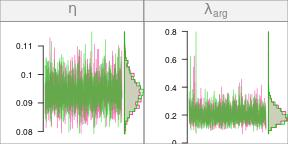### Posterior predictive fits

Once the fit is completed (and provided that no issues arised during the Stan run), we can calculate the posterior predicted trajectories and check if they match with the observed data:

pred <- predict(m, run, draws = 200)
plot(pred, facet_row = "group", facet_col = "compartment", type = "prop",
scale = "all", log = TRUE,
comps = c("epi", "FBOM", "petro", "pseph", "tricor", "arg"))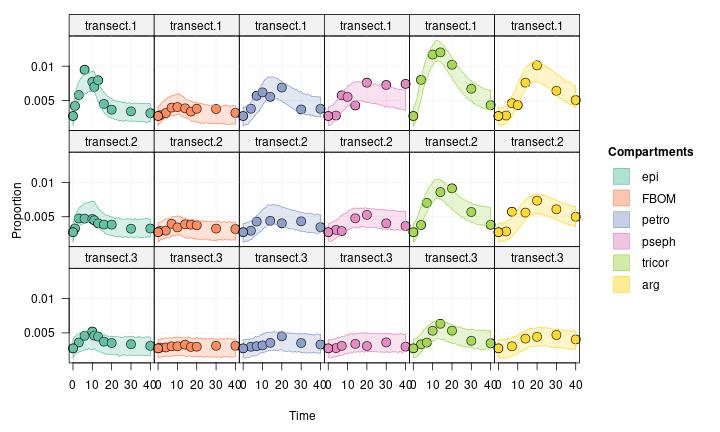The quality of the fit looks quite good. We could use this fit to estimate flows between compartments and make quantitative conclusions from those results. We can also use this model as a base model to test for putative trophic link.

For example, one putative link is that Argia might feed on Psephenus in addition to feeding on Petrophila and Tricorythodes. We can build an run an alternative model with this link, and compare the two models.

## Alternative model

The data used for this alternative model is the same, only the topology of the model is changed:

m2 <- m %>% set_topo("NH4, NO3 -> epi, FBOM", "epi -> petro, pseph",
"FBOM -> tricor", "petro, pseph, tricor -> arg")

Changing the topology resets steady and split compartments and priors. Let’s apply the same settings as for the first model:

m2 <- m2 %>%
set_split(c("epi", "FBOM"))

The structure of the alternative model is:

ggtopo(m2, "sugiyama")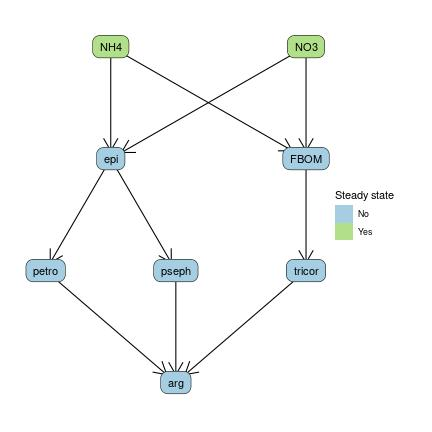Let’s apply the same priors as for the first model:

m2 <- set_priors(m2, priors(m))

Do we have any new prior we have to set in m2?

missing_priors(m2)
## # A tibble: 1 × 2
##   in_model             prior
##   <chr>                <list>
## 1 upsilon_pseph_to_arg <NULL>

Let’s use the same prior as for the other upsilon_* rates from biological compartments:

m2 <- set_priors(m2, normal_p(0, 0.5), "upsilon_pseph_to_arg")

We run the model:

run2 <- run_mcmc(m2, iter = 2000)
plot(run2)
# Note: the figure below only shows a few of the traceplots for vignette concision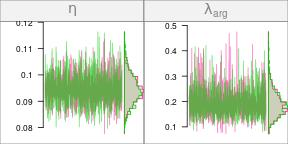We check the model fit with a posterior predictive fit:

pred2 <- predict(m2, run2, draws = 200)
plot(pred2, facet_row = "group", facet_col = "compartment", type = "prop",
scale = "all", log = TRUE,
comps = c("epi", "FBOM", "petro", "pseph", "tricor", "arg"))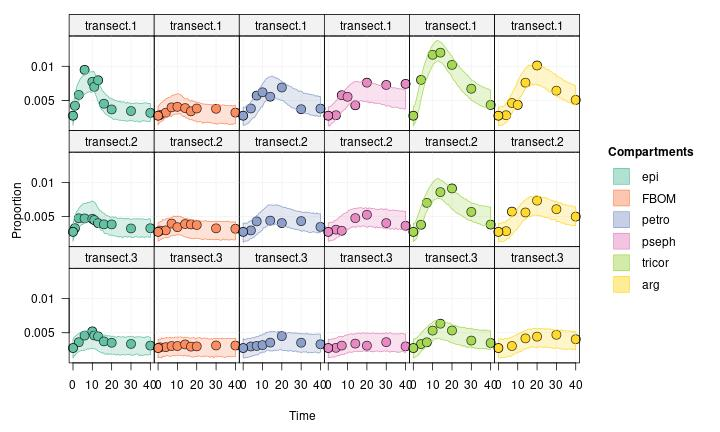When comparing with the first fit, this one looks equally good as before. Which model should we prefer?

## Model comparison

We compare the two models by calculating their DIC and choosing the model with the lowest DIC.

Note that the use of DIC is not ideal here: DIC is valid when the posteriors have a multinormal distribution, which is not the case here given that the parameters are bounded by zero and several exhibit skewness.

An alternative to DIC is to use LOO (leave-one-out validation). However, LOO requires independent data, and the data used here is a time series where data points are not independent.

We use the dic() function to calculate the model DIC values and the corresponding DIC weight:

dic(run, run2)
## # A tibble: 2 × 6
##   fit     Dbar    pD    DIC delta_DIC weight
##   <chr>  <dbl> <dbl>  <dbl>     <dbl>  <dbl>
## 1 run   -1821.  22.9 -1798.      0    0.907
## 2 run2  -1819.  25.4 -1794.      4.56 0.0928

run is from the model fit without the trophic link pseph -> arg. According to the DIC, this model is more likely than the alternative model with the extra trophic link. The evidence is reasonable but not overwhelming, as evidenced by the $$\Delta$$DIC value and the DIC weights.

## Sankey plot

Let’s estimate the flows between compartments. Since this network has steady state sources, we can estimate flows at equilibrium:

flows <- tidy_flows(m, run, n = 500, steady_state = TRUE)

Sankey plot:

f <- flows %>%
select(flows) %>%
unnest(flows) %>%
na.omit() %>% # We drop the rows corresponding to lambda losses
group_by(from, to) %>%
summarize(flow = mean(average_flow),
sd = sd(average_flow),
cv = sd / flow)
## summarise() has grouped output by 'from'. You can override using the .groups
## argument.
nodes <- inits %>%
ungroup() %>%
filter(transect == "transect.1") %>%
select(comp = compartment, size = mgN.per.m2) %>%
mutate(label = comp)
sankey(topo(m), nodes, f %>% select(from, to, flow) %>% rename(width = flow),
edge_f = 0.2, layout = "left2right")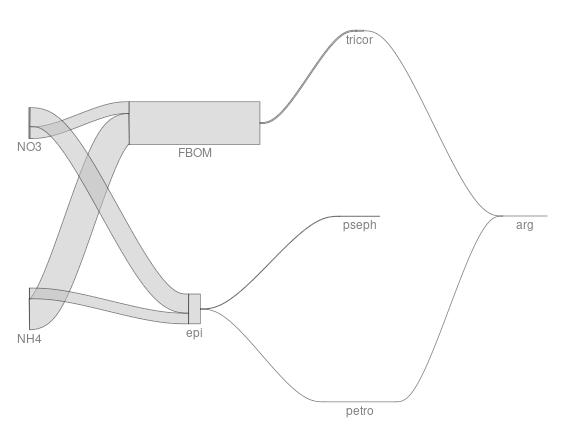## To go further

A heatmap of the correlation between parameter estimates can be a good way to visualize the strength of the interdependence between parameters:

mcmc_heatmap(run)